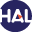Home page > 03. Volume 2 N°1 (2005) > On the numerical simulation of multiphase water flows with changes of phase (...)

# On the numerical simulation of multiphase water flows with changes of phase and strong gradients using the Homogeneous Equilibrium Model

Tuesday 4 January 2005,

Full Title: On the numerical simulation of multiphase water flows with changes of phase and strong gradients using the Homogeneous Equilibrium Model

Abstract:

We introduce a general method based on a variant of the Flux Characteristic method described by Ghidaglia et al  designed to simulate water-vapour two-phase flows. As an example, we use the three equations Homogeneous Equilibrium model (HEM) with hypotheses of local thermodynamic equilibrium. Our purpose here is to analyze the Finite Volume method when strong gradients of density are present and when some derivatives of quantities like speed of sound strongly vary due to phase transitions. As framework for numerical experiments, we consider a complex flow inside an injector-condenser device. The analysis will lead to a variant of the Flux Characteristic method with regularized matrix-valued sign functions. Other applications like water boiling into a hot channel and a fall of pressure in a crack due to friction will be also considered.

Date of publication : January 2005Voir sur HAL : https://hal.archives-ouvertes.fr/hal-01123347